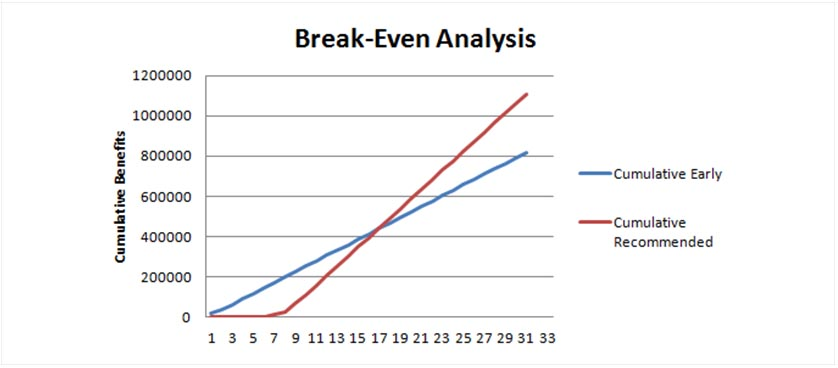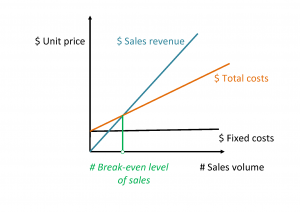Get the opportunity to grow your influence by giving your products or services prime exposure with Performance Magazine.

Our Websites

# When marketing meets finance: the break-even level of salesIn a previous blog post we investigated the Return on Marketing Investment, a metric that analyzes the marketing activity from a financial point of view that is more complex than the revenue from sales generated by marketing. The current blog post aims at exploring another area where finance meets marketing: the break-even analysis. In this area, we consider that finance actually needs marketing: the break-even analysis aims at determining from which point on (i.e. volume of sales), a business begins to generate profits. Calculating the break-even level of sales is done by following the steps below:

• Calculate your fixed production costs. These include the costs to produce that do not vary upon the volume of production and generally include the administrative costs (rent, secretary etc.)
• Calculate the variable unit costs. These are costs that depend on the production volume (such as: salaries for the operational employees, utilities in the plant, raw materials etc.), that are divided to the volume of production to obtain the cost per unit.
• Estimate the price that will be charged for each unit of the product or service. There are various price-setting strategies, such as adding a margin to the production costs, considering competition prices and even the break-even analysis itself can be used at setting the right price.

The break-even level of sales is then calculated as:

# Break-even level of sales = \$ Fixed costs / (\$ Price per unit – \$ Unit variable cost)

The calculation formula is derived by the equation that reflects the zero profits:

\$ Total revenue = \$ Total costs

The result indicates the sales volume that needs to be achieved in order to attain the break-even – at which the revenues cover the costs, but no profits are obtained. From this sales level on, the business begins to generate profits.After having calculated the break-even level of sales based on mostly financial inputs, the marketing department can estimate when and whether these levels of sales can be achieved and in which conditions. Thus, the contribution of the break-even level of sales is considerable in what regards several financial decisions:

• Finding the optimal fixed to variable costs combination
• Comparing the estimated profitability of various options for investment
• Setting optimum price levels

To take the analysis a step further, finance managers can even set targets for the profits they intend to achieve and calculate the expected level of sales that would generate these profits. This is done by adjusting the break-even calculation to include the profit targets, meaning we will now calculate the level of sales that will generate an expected amount of profits, not zero profits (as in the case of the break-even level of sales):

# Target volume = (\$ Fixed costs + \$ Profit) / (\$ Price per unit – \$ Unit variable cost)

For further reading and examples of calculating the break-even level of sales, you can follow: Break-Even Analysis, Weatherhead School of Management David Kinard (2009), Metric Monday – How Much is that Marketing Effort Worth?

Image Source: